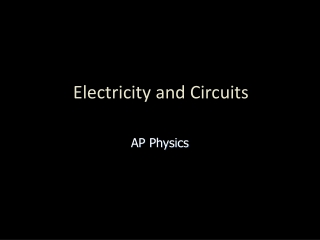DownloadDownload PresentationElectricity and Circuits

# Electricity and Circuits

Télécharger la présentation## Electricity and Circuits

- - - - - - - - - - - - - - - - - - - - - - - - - - - E N D - - - - - - - - - - - - - - - - - - - - - - - - - - -
##### Presentation Transcript

1. Electricity and Circuits AP Physics

2. +Q + A t Wire - Electric Current Electric currentI is the rate of the flow of charge Q through a cross-section A in a unit of time t. One ampere A is charge flowing at the rate of one coulomb per second.

3. I = 6 A Example 1. The electric current in a wire is 6 A. How many electrons flow past a given point in a time of 3 s? q = (6 A)(3 s) = 18 C Recall that: 1 e- = 1.6 x 10-19 C, then convert: In 3 s: 1.12 x 1020 electrons

4. + - + - + + - - Electron flow Conventional flow + - e- + Conventional Current Imagine a charged capacitor with Q = CV that is allowed to discharge. Electron flow: The direction of e- flowing from – to +. Conventional current: The motion of +q from + to – has same effect. Electric fields and potential are defined in terms of +q, so we will assume conventional current (even if electron flow may be the actual flow).

5. Power lines Battery Wind generator Electromotive Force A source of electromotive force (emf) is a device that uses chemical, mechanical or other energy to provide the potential difference necessary for electric current.

6. High potential Low potential Constriction Resistor High pressure Low pressure + - R I Switch Water Flow Valve Source of EMF Water Pump Water Analogy to EMF The source of emf (pump) provides the voltage (pressure) to force electrons (water) through electric resistance (narrow constriction).

7. Ground Battery Resistor + - + - + - - + - + - Electrical Circuit Symbols Electrical circuits often contain one or more resistors grouped together and attached to an energy source, such as a battery. The following symbols are often used:

8. Steel Copper Glass Is Ic Ig 4 V 4 V 4 V Electric Resistance Suppose we apply a constant potential difference of 4 V to the ends of geometrically similar rods of, say: steel, copper, and glass. The current in glass is much less than for steel or iron, suggesting a property of materials called electrical resistance R.

9. Ohm’s Law Ohm’s law states that the current I through a given conductor is directly proportional to the potential difference V between its end points. Ohm’s law allows us to define resistance R and to write the following forms of the law:

10. + - R I 6 mA V = 3 V Source of EMF Example 2. When a 3-V battery is connected to a light, a current of 6 mA is observed. What is the resistance of the light filament? R = 500 W The SI unit for electrical resistance is the ohm, W:

11. A + Rheostat Emf V - Source of EMF Rheostat Voltmeter Ammeter Laboratory Circuit Symbols

12. L 2L 1 W 2 W 2A A 2 W 1 W Factors Affecting Resistance 1. The length L of the material. Longer materials have greater resistance. 2. The cross-sectional area A of the material. Larger areas offer LESS resistance.

13. R > Ro Ro Ri > Rc Copper Iron Factors Affecting R (Cont.) 3. The temperature T of the material. The higher temperatures usually result in higher resistances. 4. The kind of material. Iron has more electrical resistance than a geometrically similar copper conductor.

14. Resistivity of a Material The resistivity r is a property of a material that determines its electrical resistance R. Recalling that R is directly proportional to length L and inversely proportional to area A, we may write: The unit of resistivity is the ohm-meter (W·m)

15. Example 3.What lengthL of copper wire is required to produce a 4 mW resistor? Assume the diameter of the wire is 1 mm and that the resistivity r of copper is 1.72 x 10-8 W.m. A = 7.85 x 10-7 m2 L = 0.183 m Required length is:

16. V q I V Electric Power Electric powerP is the rate at which electric energy is expended, or work per unit of time. To charge C: Work = qV Substitute q = It , then: P = VI

17. Calculating Power Using Ohm’s law, we can find electric power from any two of the following parameters: current I, voltageV, and resistanceR. Ohm’s law: V = IR

18. Example 5.A power tool is rated at 9 A when used with a circuit that provides 120-V. What power is used in operating this tool? P = VI = (120 V)(9 A) P = 1080 W Example 6.A 500-W heater draws a current of 10 A. What is the resistance? R = 5.00 W

19. Summary of Formulas Electric current: Ohm’s Law

20. Summary (Cont.) Resistivity of materials: Electric Power P: